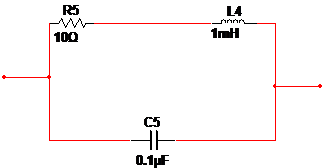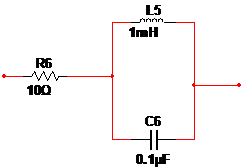### Create an Account

Home / Questions / Redraw the following schematics with the impedance of each of the element shown in Laplace...

# Redraw the following schematics with the impedance of each of the element shown in Laplace domain Then determine the overall impedance of the entire circuit between the two ends of the shown circuit

Redraw the following schematics with the impedance of each of the element shown in Laplace domain. Then determine the overall impedance of the entire circuit between the two ends of the shown circuit and express it in Laplace domain as a ratio of two polynomials in s, with the coefficients of the highest power if s in the numerator and denominator are made unity. (Follow the method outlined in the lecture to determine the impedances of elements in Laplace domain and then use the formulas for combining impedances in series and parallel.)Redraw the following schematics with the impedance of each of the element shown in Laplace domain. Then determine the overall impedance of the entire circuit between the two ends of the shown circuit and express it in Laplace domain as a ratio of two polynomials in s, with the coefficients of the highest power if s in the numerator and denominator are made unity. (Follow the method outlined in the lecture to determine the impedances of elements in Laplace domain and then use the formulas for combining impedances in series and parallel.)

Will not award for taking from another post and reposting here.View less »

Jul 15 2020 View more View LessSubscribe To Get Solution